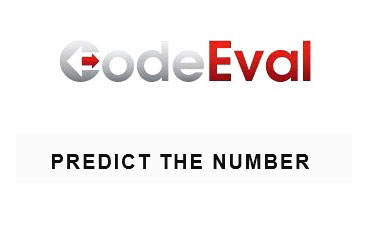# CodeEval 1 – Predict The Number

July 23, 2014Starting from this post I would like to establish another series of posts that will be different than the typical engineering/Excel/VBA posts that are being published in this blog. The purpose of this series is to demonstrate possible solutions to various CodeEval programming challenges. Each solution has already been submitted and accepted as valid on the CodeEval platform, so if you try to submit the presented solution as it is, you will probably get a “not unique solution” result. The solutions will be presented in the C# language, but the logic/algorithm behind them is similar despite the language you might use.

### What is CodeEval?

CodeEval is a platform used by developers to showcase their skills. Developers can participate in app building competitions and win cash/prizes. They can also solve programming challenges as a way to impress employers with their technical skills. Employers can use CodeEval as a way to enhance their brand by launching competitions/programming challenges and as a means to get introduced to the best developers.

### Predict the number – challenge description

Sequence 011212201220200112 … constructed as follows: first is 0, then repeated the following action: already written part is attributed to the right with replacement 0 to 1, 1 to 2, 2 to 0. E.g.

0 -> 01 -> 0112 -> 01121220 -> …

Create an algorithm that determines what number is on the N-th position in the sequence.

#### Input sample

Your program should accept as its first argument a path to a filename. Each line in this file contains an integer N such as 0 <= N <= 3000000000. Example:

0
5
101
25684

#### Output sample

Print out the number which is on the N-th position in the sequence. Example:

0
2
1
0

The particular challenge has a quite low success rate (59.8% – 23/7/2014) and its level of difficulty is medium. More info you can find here (update: the CodeEval has shut down, so the link was removed).

### Solution

For this challenge I tried at least 3 different solutions but, all of them failed due to the time limitation (the code you submit must complete the given test case without errors and within a certain amount of time – a few ms). Finally, after spending some time I discovered the required pattern and the working solution is given below:

``````using System;
using System.Collections.Generic;
using System.IO;
using System.Linq;
using System.Text;

/*
-------------------------------------------------------------------
The code below solves the CodeEval challenge - Predict the number.

Written by:    Christos Samaras
Date:          28/06/2014
e-mail:        [email protected]
site:          http://www.myengineeringworld.net
-------------------------------------------------------------------
*/

namespace PredictTheNumber
{
class Program
{
static void Main(string[] args)
{
{
if (line != null)
{
long numberToFind = long.Parse(line);
int cnt = 0;
bool done = false;

if (numberToFind > 0)
{
for (int i = 0; i < 33; i++)
{
if (numberToFind < (long)Math.Pow(2, i))
{
long result = 0;
long previous = 0;

for (int j = i - 1; j > -1; j--)
{
result = numberToFind - previous - (long)Math.Pow(2, j);
if (result == 0)
{
cnt++;
done = true;
break;
}
if (result == 1)
{
cnt += 2;
done = true;
break;
}
if (result > 0)
{
cnt++;
previous += (long)Math.Pow(2, j);
}
if (j == 0)
{
done = true;
break;
}
}
}
if (done)
break;
}
Console.WriteLine((cnt % 3).ToString());
}
else
Console.WriteLine("0");
}
}
}
}
}

/* NOTE: the solution has already been submitted and accepted as valid! */
``````

### Points

Here is proof that the solution works and the points given by the CodeEval platform.So, what do you think? Do you find this kind of problem interesting or not? Write your thoughts in the comments below.

Category: CodeEval